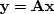A great many of the calculations we do in science and engineering are really matrix multiplication in disguise. The first goal of this chapter is to unmask the disguise by showing many examples. Second, we will illuminate the meaning of the adjoint operator (matrix transpose) in these many examples.

Geophysical modeling calculations generally use linear operators that predict data from models. Our usual task is to find the inverse of these calculations, i.e., to find models (or make maps) from the data. Logically, the adjoint is the first step and a part of all subsequent steps in this inversion process. Surprisingly, in practice the adjoint sometimes does a better job than the inverse! This is because the adjoint operator tolerates imperfections in the data and does not demand that the data provide full information.

Using the methods of this chapter, you will find that once you grasp the relationship between operators in general and their adjoints, you can have the adjoint just as soon as you have learned how to code the modeling operator.

If you will permit me a poet's license with words, I will offer you the following table of operators and their adjoints:


matrix multiply 		conjugate-transpose matrix multiply
convolution 		crosscorrelation
stretching 		squeezing
causal integration 		anticausal integration
plane-wave superposition 		slant stack / beam forming
superposing on a curve 		summing along a curve
upward continuation 		downward continuation
diffraction modeling 		imaging by migration
hyperbola modeling 		CDP stacking
ray tracing 		tomography


The left column above is often called modeling," and the adjoint operators on the right are often used in data processing."

When the adjoint operator is not an adequate approximation to the inverse, then you apply the techniques of fitting and optimization which require iterative use of the modeling operator and its adjoint.

The adjoint operator is sometimes called the back projection" operator because information propagated in one direction (earth to data) is projected backward (data to earth model). With complex-valued operators the transpose and complex conjugate go together and in Fourier analysis, taking the complex conjugate ofreverses the sense of time. Still assuming poetic license, I will say that adjoint operators undo the time and phase shifts of modeling operators. The inverse operator does this too, but it also divides out the color. For example, with linear interpolation high frequencies are smoothed out, so inverse interpolation must restore them. You can imagine the possibilities for noise amplification. That is why adjoints are safer than inverses. But nature determines in each application what is the best operator to use, whether to stop after the adjoint, to go the whole way to the inverse, or to stop part-way.

We will see that computation of the adjoint is a straightforward adjunct to the computation itself, and the computed adjoint should be, and generally can be, exact (within machine precision). If the application's operator is computed in an approximate way, we will see that it is natural and best to compute the adjoint with adjoint approximations.

Much later in this chapter is a formal definition of adjoint operator. Throughout the chapter we handle an adjoint operator as a matrix transpose, but we hardly ever write down any matrices or their transposes. Instead, we always prepare two subroutines, one that performsand another that performs,so we need a test that the two subroutines really embody the essential aspects of matrix transposition. Although the test is an elegant and useful test and is itself a fundamental definition, curiously, that definition helps us not one bit in constructing adjoint operators, so I postpone the formal definition of adjoint until after we have seen many examples.